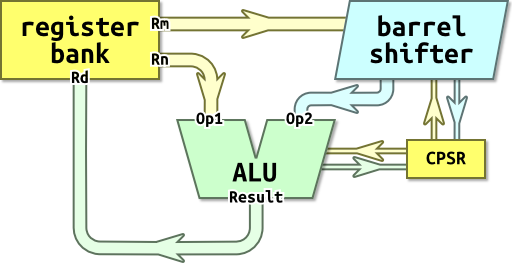ARM Instruction Set
• ARM Instruction Set• PSR(Program Status Register)• Condition field
• −x = not(x)+1 and computes a−b as a+not(b)+1.
• The carry flag is set according to this addition.
• C set = higher or the same
• The same: 1-1=1+not(1)+1=00000001+11111110+1=1(carry set), 00000000
• Lower: 1-2=1+not(2)+1=00000001+11111101+1=0(carry clear), 11111111
• Arithmetic Operations
 SBC r0, r1, r2 r0 := r1-r2+C-1 The SBC (Subtract with Carry) instruction subtracts the value of Operand2 from the value in Rn. If the carry flag is clear, the result is reduced by one.
• Barrel Shifter
• Multiplication Implementation
• Addressing
````LDR R0, [R1, R2]`
```• Stack
• Ascending vs. Descending
• Full v.s. Empty
• DMB ensures that all explicit memory accesses before the DMB instruction complete before any explicit memory accesses after the DMB instruction start.
• This ensures correct ordering between two memory accesses.
page revision: 26, last edited: 11 Apr 2014 01:35
Unless otherwise stated, the content of this page is licensed under Creative Commons Attribution-ShareAlike 3.0 License